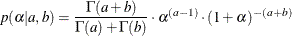### Specifying a New Distribution

To work with a new density that is not listed in the section Standard Distributions, you can use the GENERAL and DGENERAL functions. The letter “D” stands for discrete. The new distributions have to be specified on the logarithm scale.

Suppose you want to use the inverse-beta distribution:The following statements in PROC MCMC define the density on its log scale:

a = 3; b = 5;
const = lgamma(a + b) - lgamma(a) - lgamma(b);
lp = const + (a - 1) * log(alpha) - (a + b) * log(1 + alpha);
prior alpha ~ general(lp);


The symbol lp is the expression for the log of an inverse-beta (a = 3, b = 5). The function general(lp) assigns that distribution to alpha. The constant term, const, can be omitted because the Markov simulation requires only the log of the density kernel.

You can use the GENERAL function to specify a distribution for a single variable or for multiple variables. It is important to emphasize that the argument lp is an expression for the log of the joint distribution for these variables. On the contrary, any standard distribution is applied separately to each random variable in the statement.

When you use the GENERAL function in the MODEL statement, you do not need to specify the dependent variable on the left of the tilde ~. The log-likelihood function takes the dependent variable into account; hence, there is no need to explicitly state the dependent variable in the MODEL statement. However, in the PRIOR and RANDOM statements, you need to explicitly state the parameter names and a tilde with the GENERAL function.

You can specify any distribution function by using the GENERAL and DGENERAL functions as long as the distribution function is programmable with SAS statements. When the function is used in the PRIOR statements, you must supply initial values in either the PARMS statement or within the BEGINCNST and ENDCNST statements. See the sections PARMS Statement and BEGINCNST/ENDCNST Statement. When the function is used in the RANDOM statement, you must use the INITIAL= option in the RANDOM statement to supply initial values

Note: PROC MCMC does not verify that the GENERAL function you specify is a valid distribution—that is, an integrable density. You must use the function with caution.